# High School Math : Quadrilaterals

## Example Questions

1 2 3 4 5 6 7 8 9 10 12 Next →

### Example Question #2 : How To Find The Length Of The Side Of A Square

When the side of a certain square is increased by 2 inches, the area of the resulting square is 64 sq. inches greater than the original square.  What is the length of the side of the original square, in inches?

18

15

14

17

16

15

Explanation:

Let x represent the length of the original square in inches.  Thus the area of the original square is x2.  Two inches are added to x, which is represented by x+2.  The area of the resulting square is (x+2)2.  We are given that the new square is 64 sq. inches greater than the original.  Therefore  we can write the algebraic expression:

x2 + 64 = (x+2)2

FOIL the right side of the equation.

x2 + 64 = x2 + 4x + 4

Subtract xfrom both sides and then continue with the alegbra.

64 = 4x + 4

64 = 4(x + 1)

16 = x + 1

15 = x

Therefore, the length of the original square is 15 inches.

If you plug in the answer choices, you would need to add 2 inches to the value of the answer choice and then take the difference of two squares.  The choice with 15 would be correct because 172 -152 = 64.

### Example Question #3 : How To Find The Length Of The Side Of A Square

If the area of a square is 25 inches squared, what is the perimeter?

25

15

20

10

Not enough information

20

Explanation:

The area of a square is equal to length times width or length squared (since length and width are equal on a square). Therefore, the length of one side is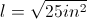or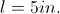The perimeter of a square is the sum of the length of all 4 sides or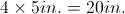### Example Question #4 : How To Find The Length Of The Side Of A Square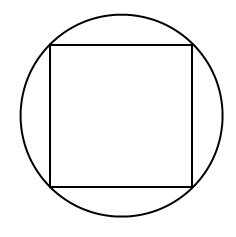A square is inscribed inside a circle, as illustrated above. The radius of the circle is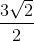units. If all of the square's diagonals pass through the circle's center, what is the area of the square?units squared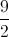units squared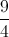units squaredunits squaredunits squaredunits squared

Explanation:

Given that the square's diagonals pass through the circle's center, those diagonals must each form a diameter of the circle. The circle's diameter is twice its radius, i.e.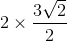, which is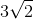. Since this diameter (i.e., the square's diagonal) is the hypotenuse of a right triangle formed by two sides of the square, the length of one of the square's sides can be calculated with the Pythagorean Theorem.replaceandbecause the sides of the square must be equal in length. Since the objective is to solve for the square's area, solve for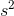since one side squared will be the square's area.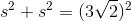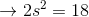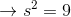units squared

### Example Question #1 : Quadrilaterals

Quadrilateral ABCD contains four ninety-degree angles. Which of the following must be true?

I. Quadrilateral ABCD is a rectangle.

II. Quadrilateral ABCD is a rhombus.

III. Quadrilateral ABCD is a square.

I, II, and III

I and II only

I only

II only

II and III only

I only

Explanation:

Quadrilateral ABCD has four ninety-degree angles, which means that it has four right angles because every right angle measures ninety degrees. If a quadrilateral has four right angles, then it must be a rectangle by the definition of a rectangle. This means statement I is definitely true.

However, just because ABCD has four right angles doesn't mean that it is a rhombus. In order for a quadrilateral to be considered a rhombus, it must have four congruent sides. It's possible to have a rectangle whose sides are not all congruent. For example, if a rectangle has a width of 4 meters and a length of 8 meters, then not all of the sides of the rectangle would be congruent. In fact, in a rectangle, only opposite sides need be congruent. This means that ABCD is not necessarily a rhombus, and statement II does not have to be true.

A square is defined as a rhombus with four right angles. In a square, all of the sides must be congruent. In other words, a square is both a rectangle and a rhombus. However, we already established that ABCD doesn't have to be a rhombus. This means that ABCD need not be a square, because, as we said previously, not all of its sides must be congruent. Therefore, statement III isn't necessarily true either.

The only statement that has to be true is statement I.

### Example Question #111 : Quadrilaterals

A quadrilateral with congruent sides is a __________.

parallelogram

square, rectangle, or rhombus

square or rhombus

rectangle

square or rhombus

Explanation:

A rhombus is any quadrilateral with four congruent sides. A square is a quadrilateral with four congruent sides and four right angles. A square is also a rhombus.

Rectangles will have two pairs of congruent sides, but not all four sides will be congruent.

### Example Question #1 : How To Find If Rhombuses Are Similar

A quadrilateral ABCD has diagonals that are perpendicular bisectors of one another. Which of the following classifications must apply to quadrilateral ABCD?

I. parallelogram

II. rhombus

III. square

I and III only

I and II only

II and III only

I, II, and III

I and II only

Explanation:

If the diagonals of a quadrilateral are perpendicular bisectors of one another, then the quadrilateral must be a rhombus, but not necessarily a square. Since all rhombi are also parallelograms, quadrilateral ABCD must be both a rhombus and parallelogram.

### Example Question #1 : How To Find The Area Of A Rhombus

This figure is a rhombus with a side of 8 in.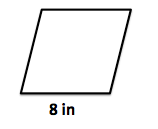What is the area of the rhombus (in2)?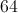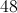Not enough information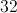Not enough information

Explanation:

In order to determine the area of a rhombus you either need a base and a height OR the length of the two diagonals OR the base and an angle.  Because we are only given the base with no information about the height, there is not enough information to determine the area of the rhombus.

### Example Question #2 : How To Find The Area Of A Rhombus

Find the area of the following rhombus: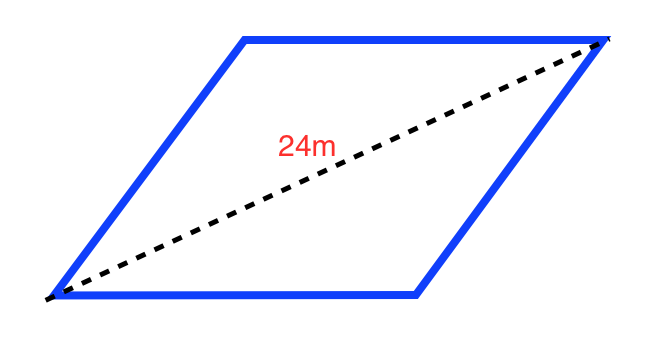The perimeter of the rhombus is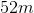.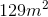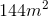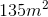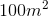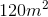Explanation:

The formula for the perimeter of a rhombus is: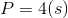Whereis the length of the side

Plugging in our values, we get: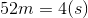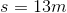The formula for the area of a rhombus is: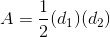Where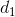is the length of one diagonal and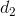is the length of another diagonal

By drawing the diagonals, we create a right triangle with the hypotenuse as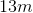and the side as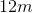.

Since we know that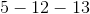is a phythagorean triple, we can infer that the third side is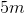.

Plugging in our values, we get: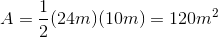### Example Question #1 : How To Find The Perimeter Of A Rhombus

This figure is a rhombus with a side of 8 in.What is the perimeter of the rhombus (in)?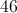Explanation:

The perimeter of the rhombus is the distance around the outside of the figure.  Since a rhombus has 4 congruent sides, the perimeter is simply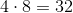.

### Example Question #678 : High School Math

You are given a rhombus with a side ofmeters. What is the perimeter of the rhombus?metersmetersmetersmetersmetersmeters
A rhombus is a quadrilateral (sided figure) withcongruent sides. The perimeter is the sum of the sides. Thus, to find the perimeter of the rhombus, we multiply the perimeter by. This gives usmeters.